Zane Blog https://www.gravatar.com/avatar/96ec5919a1de173982539e20d0fb55da 业精于勤荒于嬉，形成思毁于随 2019-08-25T13:10:11.000Z http://luojinping.com/ Jinping Luo ljp215@foxmail.com Hexo 机器学习实战 — 支持向量机 http://luojinping.com/2019/03/04/机器学习实战-—-支持向量机/ 2019-03-04T15:23:56.000Z 2019-08-25T13:10:11.000Z 要解决的问题

# 前情回顾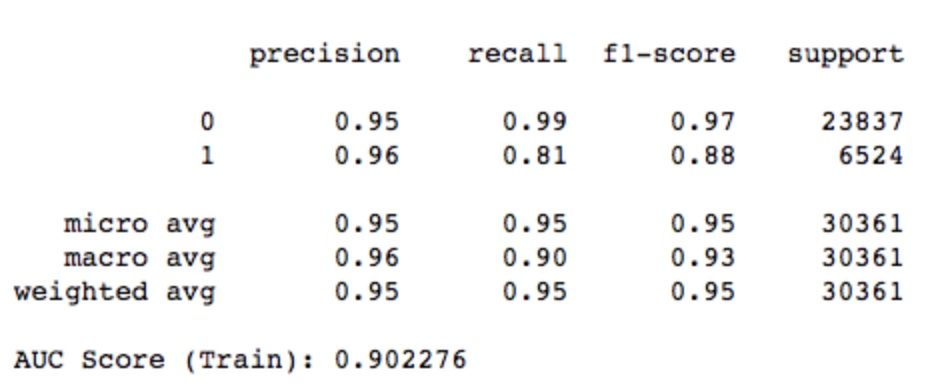# 特征工程

1. 特征选取
2. 删除无用特征
3. 空值处理
4. 处理重要特征
5. 特征标签化
6. 特征归一化

## 特征归一化

1. 模型训练速度更快
能够使参数优化时能以较快的速度收敛。
归一化前后的 SVM(linear 核) 的耗时对比，数据集 (2783, 54)，即 2783 条数据，54 个特征。归一化前后的耗时分别为：1083s，0.44s，可见归一化对计算速度的提升非常大。
2. 模型的准确率提升
将特征缩放到同一尺度的量级，能够使搜索的跳跃更加平滑，避免反复震荡的情况，提高准确率。可以参考下图形象化的解释：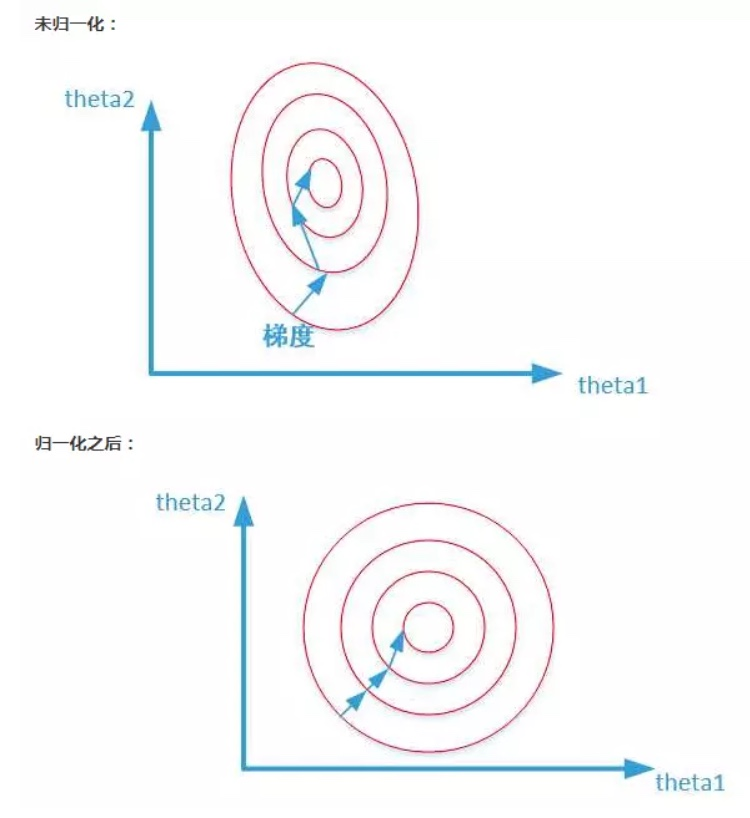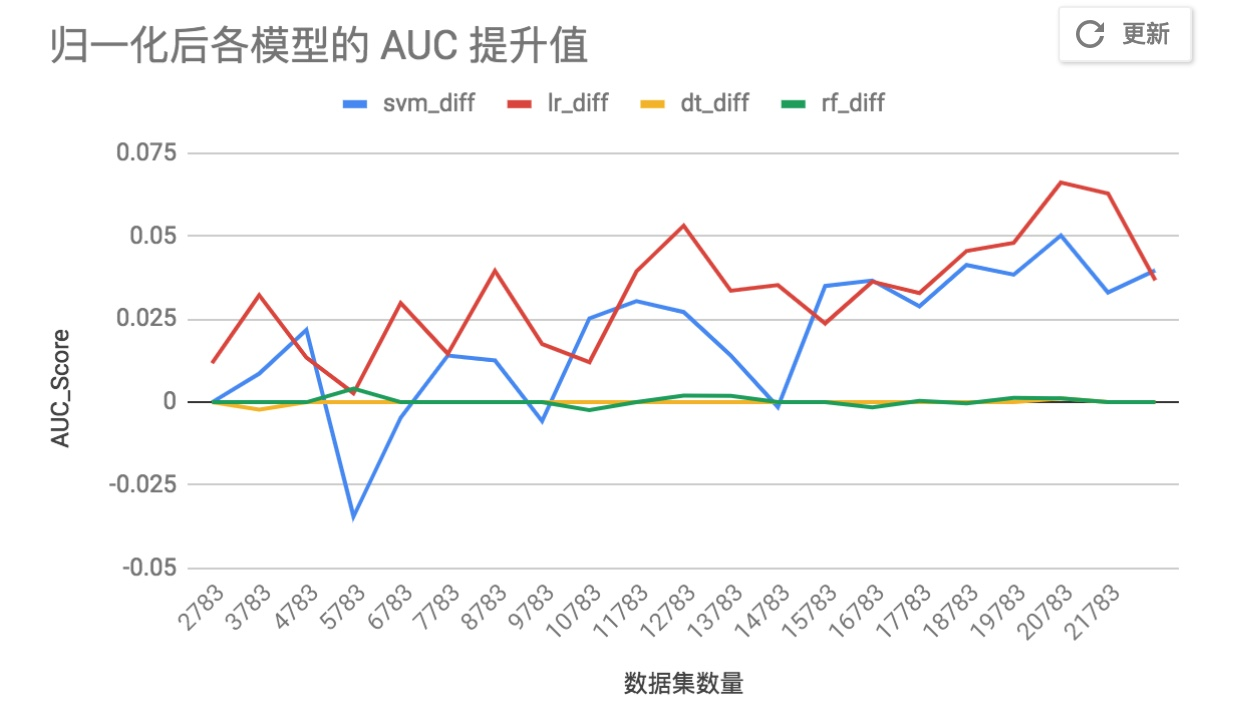# SVM

## 核函数介绍

1. linear：主要用于线性可分的情形。参数少，速度快，对于一般数据，分类效果已经很理想了。
2. rbf：将样本映射到更高维的空间，目前应用最广的一个，对大小样本都有比较好的性能，而且参数较少。linear 是 rbf 的一个特例。
3. poly：多项式核函数，将低维的输入空间映射到高纬的特征空间，参数多，当多项式的阶数比较高时，计算复杂度会非常大。
4. sigmod：支持向量机实现的就是一种多层神经网络。

Andrew Ng 的建议：

1. 如果Feature的数量很大，跟样本数量差不多，这时候选用LR或者是Linear Kernel的SVM
2. 如果Feature的数量比较小，样本数量一般，不算大也不算小，选用SVM+Gaussian Kernel
3. 如果Feature的数量比较小，而样本数量很多，需要手工添加一些feature变成第一种情况

## SVM RBF 参数搜索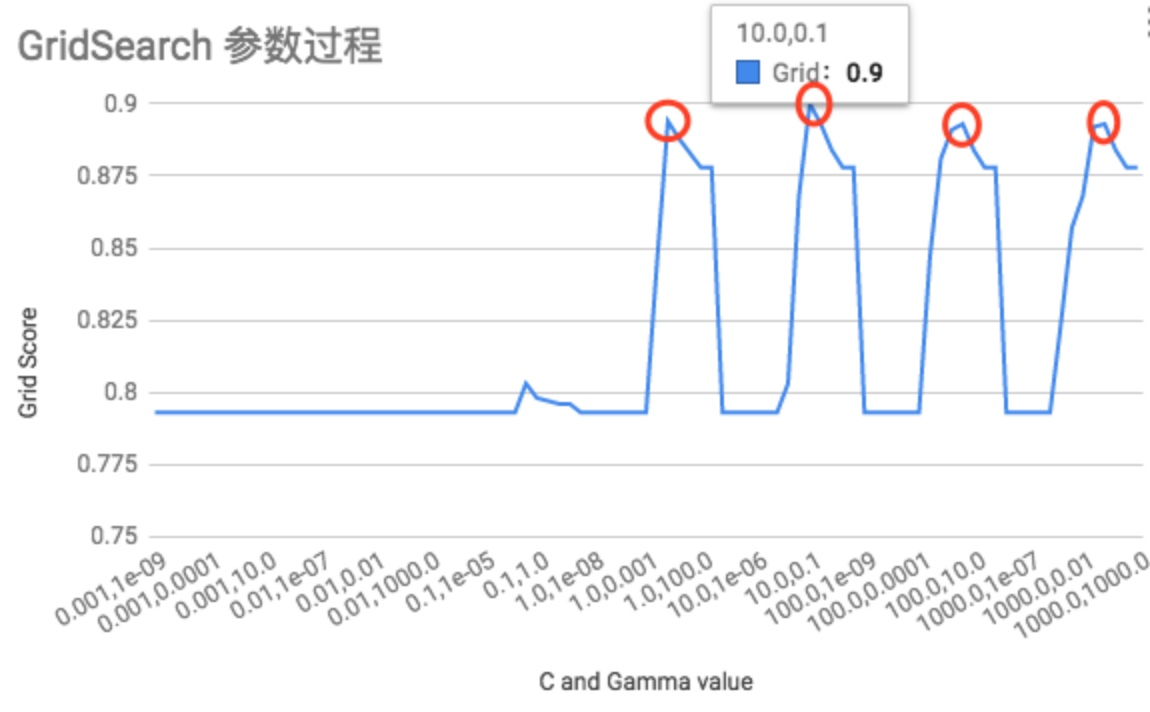C = 10, gamma = 0.1 时的效果最好。如上图中红圈所示，对于 C = 0.1, 100, 1000 时，都是gamma = 0.1 这个位置时效果最好。

## 常用核函数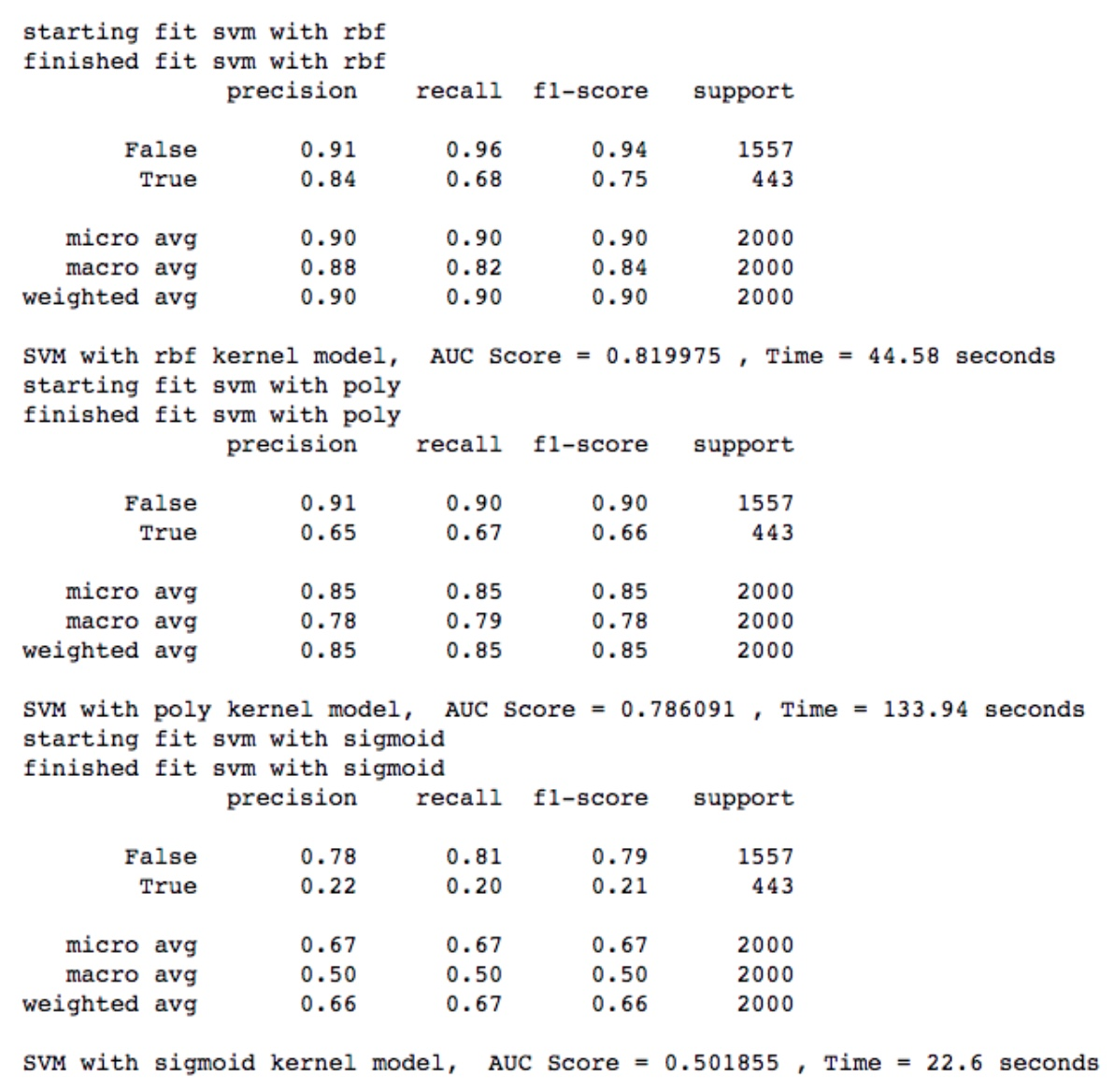## 自定义核函数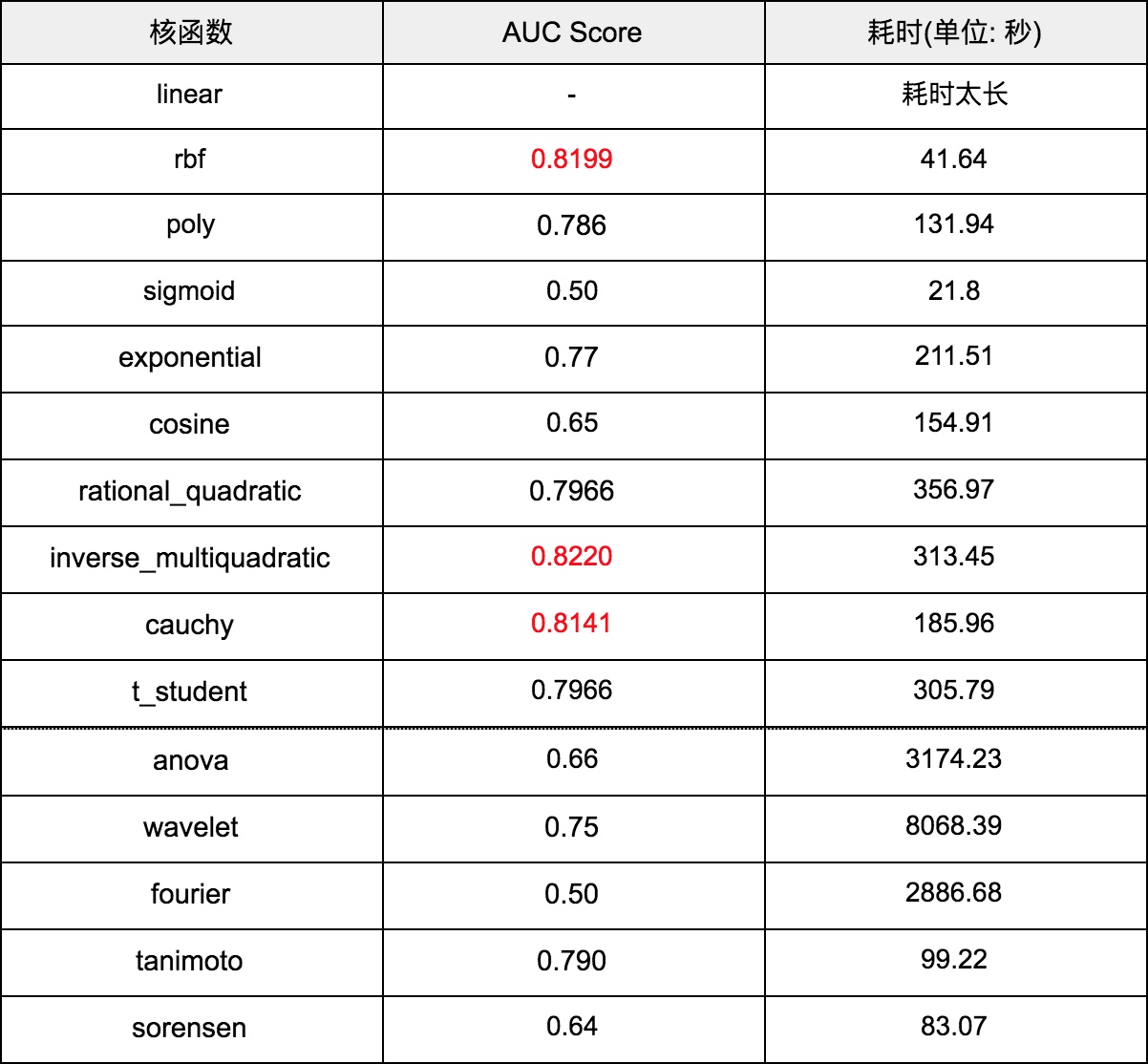# 总结

## 提升 SVM 训练速度的心得

1. 特征标签化和归一化
2. SVC 的 cache_size 设置到 7000 (M)
3. 核函数是 SVM 的关键，先用少部分数据来选核函数，再用全量数据训练
4. SVM 的 C 参数不要设置的太大

]]>

# 整体思路

• 用户被展示到该模块的埋点事件
• 用户点击该模块的埋点事件
• 用户使用过 XLJH 的统计数据
• 用户画像
• 用户的业务画像

# 特征选取

## 特征举例

• 年龄
• 性别
• 身高
• 体重
• 业务特征 1
• 业务特征 2
• 业务特征 3
• … …
• 业务特征 n-1
• 业务特征 n
• 进入首页时距离上一次使用模块的时间
• 设备机型

## 特征工程

### 删除无用特征

1. 无用特征的删除：例如 userId 等。这些特征明显与结果无关。
2. 删除由预测结果导致的特征：例如使用过该模块的时间。这些特征是在预测值为 True 时才会有值，而且这特征的赋值在要预测的事件发生之后。

### 特征的空值处理

1. 删除特征为空的数据，例如年龄，性别等必须会有的特征。
2. int 类型的特征将空值填充为 0，例如某业务的 累计分钟数，某实体数量，粉丝数量，过去7天的XX业务使用统计等
3. string 类型的特征将空值填充为 0，例如 citycode，tags 等。

### 处理重要特征

c.bmi 需要用户填入身高和体重，这部分数据缺失一些，所以填为 c.bmi 这一列的平均值，数据集中的 dataset[c.bmi].mean = 23.3174390247965，符合常识。

# 模型调优

## Decision Tree 调优

### 树的深度调优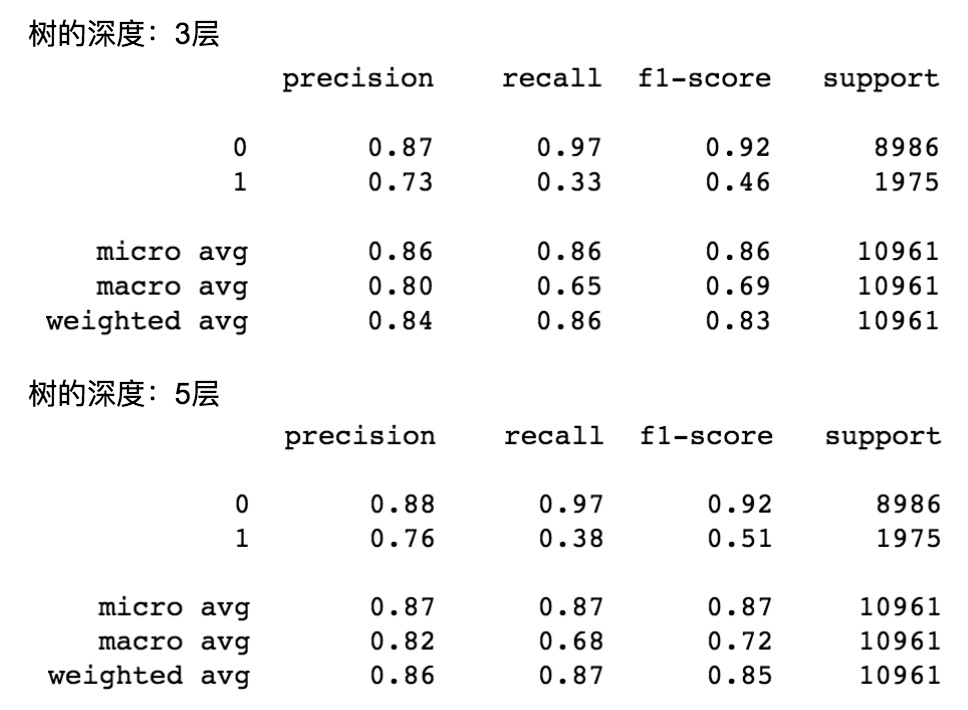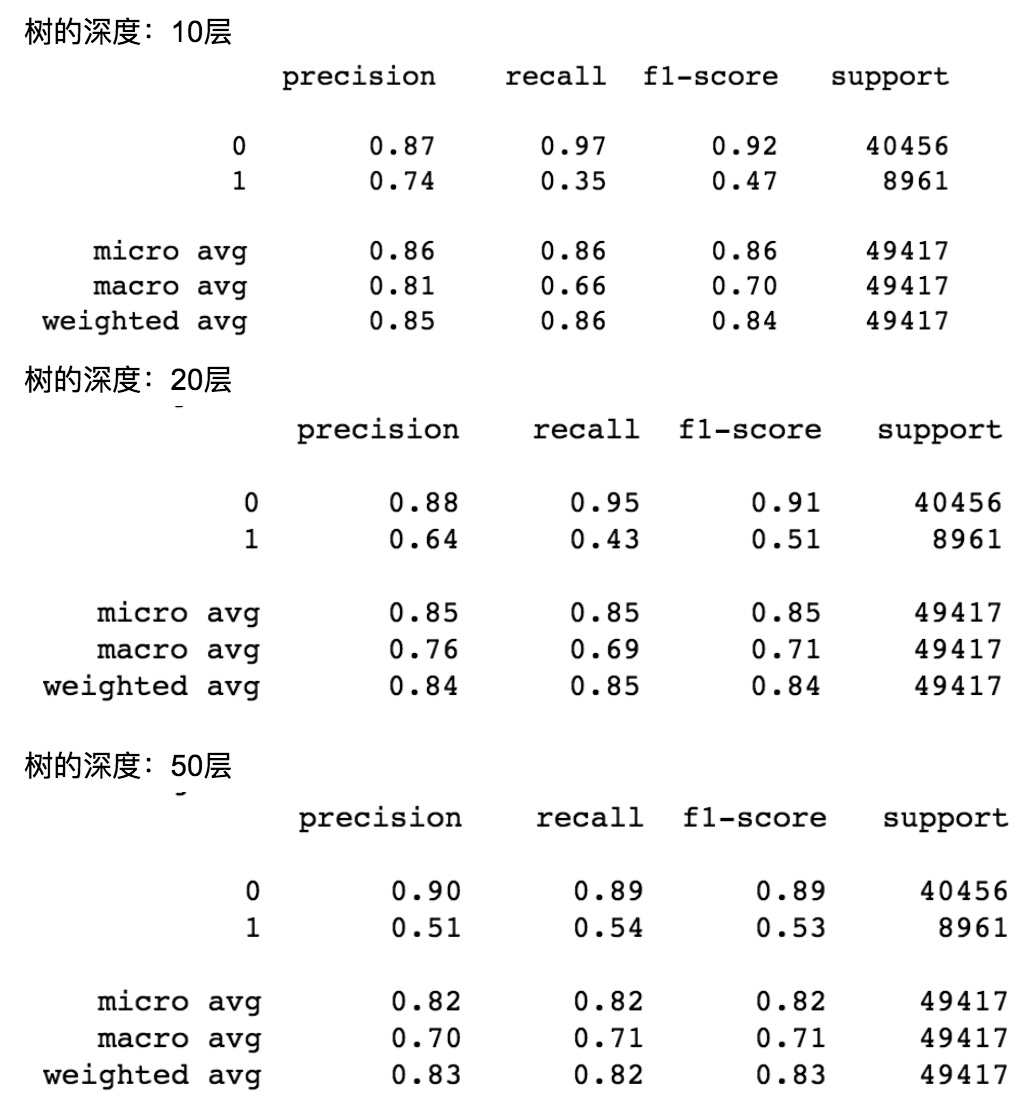### 其他参数调优

max_features=’sqrt’ 加了这个反而变差了很多。
min_samples_split=5, min_samples_leaf=5，加了这个反而变差了一些。

### DT 输出## Random Forest 调优

n_estimators：100, 300, 500 都试过，差别不大。弱学习器的最大迭代次数太小会不准确，太大模型训练地就很慢。
oob_score: True，即采用袋外样本来评估模型的好坏，提高模型拟合后的泛化能力。

Feature importances Top 10 的可视化略。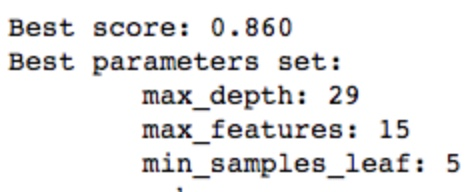learning_rate 设置为 0.02 能兼顾速度和效果。

# 踩过的坑

## PCA 降维

PCA 降维，使用 mle 和 5 个特征，都不好使，准确度反而更低，AUC 得分降至 0.51。

## 用了未来特征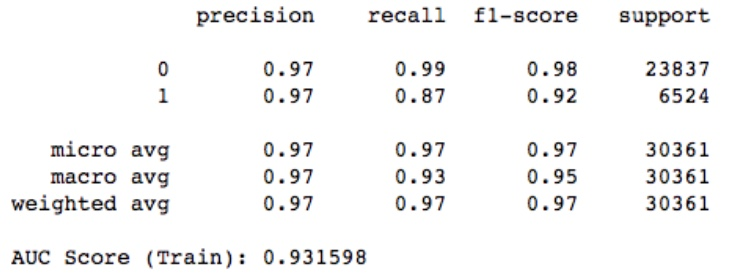# 模型对比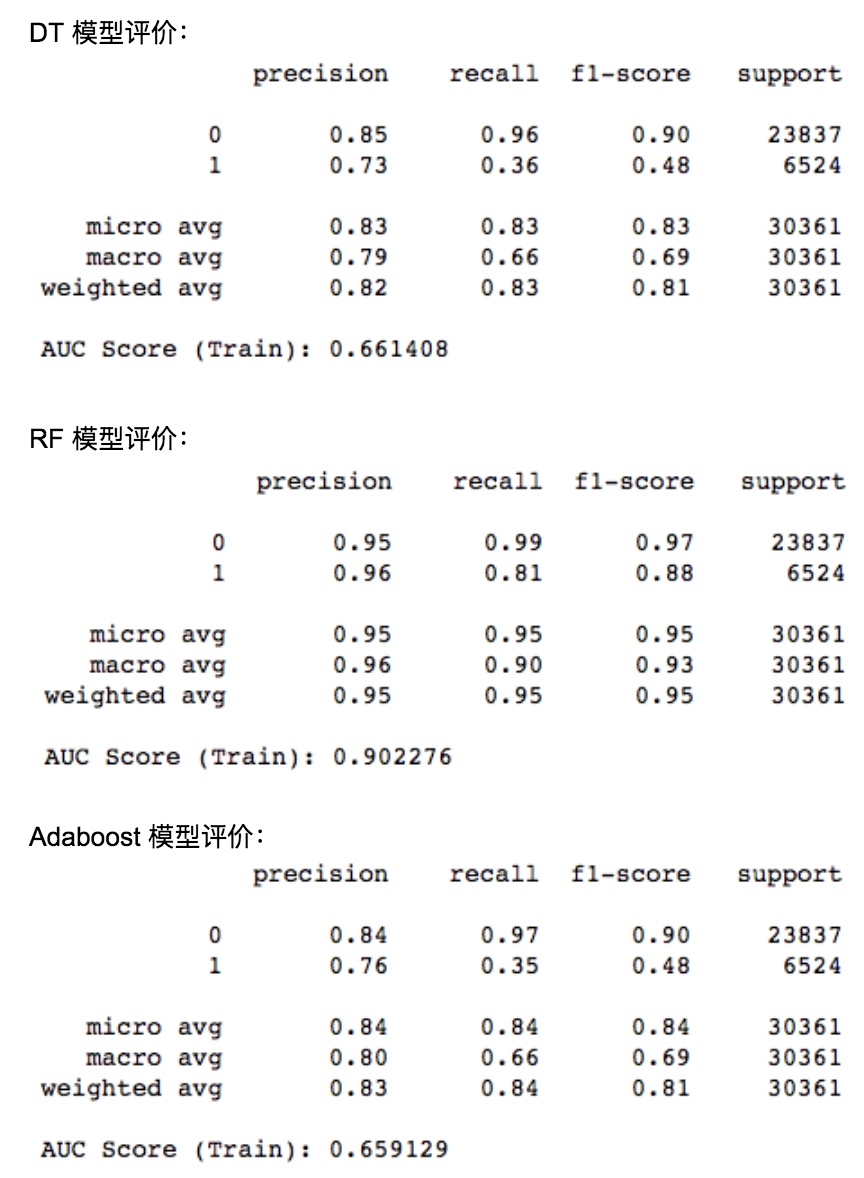RF 的准确率和召回率都还不错，感觉可以后续上线用于 App 首页了。

# 总结

1. 特征工程很重要，特征处理好后 AUC 有明显的提升
2. 调参也有用，但相比起来，好的特征更有用
3. 基尼系数和熵的区别不大
4. 树的层数越多，准确率越低，召回越高
6. gridSearchCV 搜索最有用的参数太慢了，而且最终效果还不好
7. 小心引入未来特征！

]]>

# 步骤

1. 加载数据集
2. 提取 TF-IDF 特征
3. 生成文档的 TF-IDF 矩阵
4. 训练多项式朴素贝叶斯模型
5. 评价模型

## 实现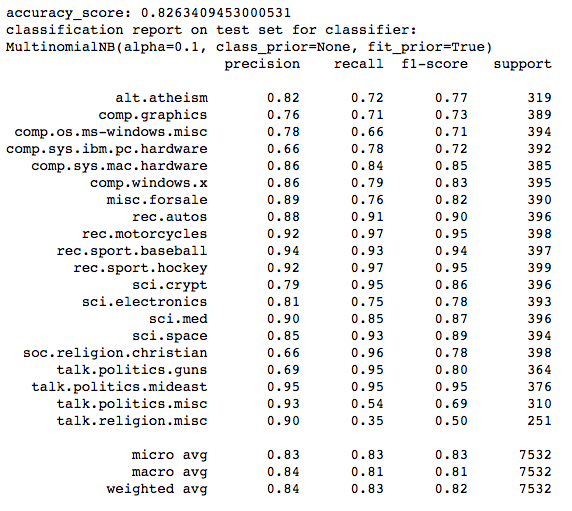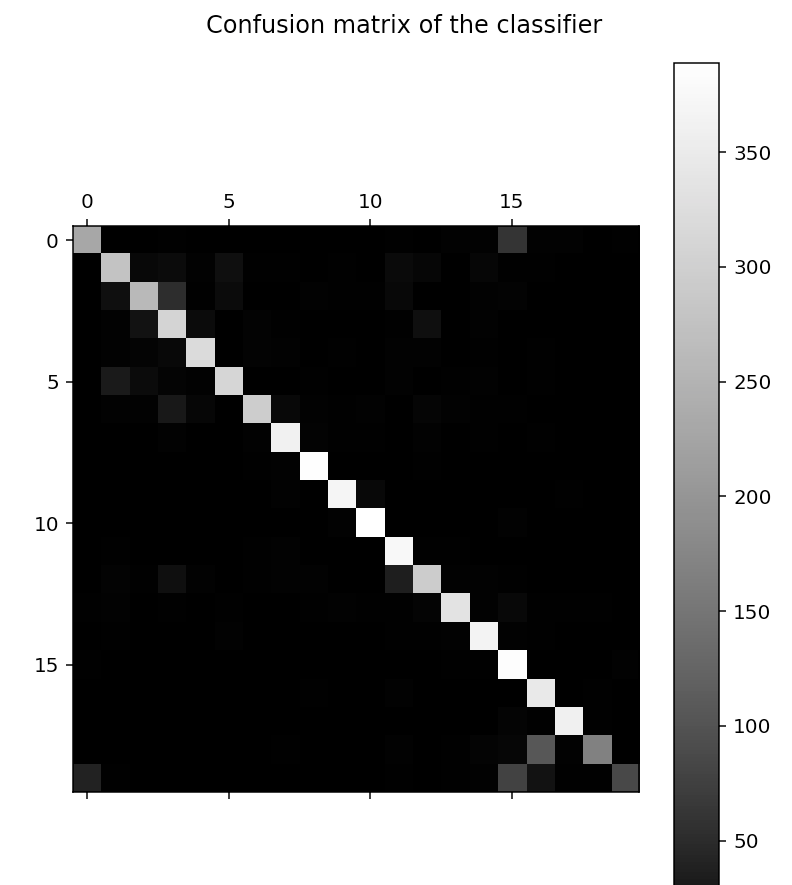# 参考文档

]]>

1. 脏数据太多，需要清洗数据
2. 增加训练数据的数量和多样性
3. 特征数量过多，使用正则化减少特征数量

# 向量的范数

L0范数：向量中非零元素的个数。
L1范数：向量中各个元素绝对值之和，又叫“稀疏规则算子”（Lasso regularization）
L2范数：向量中各个元素平方和再开方
p-范数：$||\textbf{x}||_p = (\sum_{i=1}^N|x_i|^p)^{\frac{1}{p}}$，即向量元素绝对值的p次方和的1/p次幂。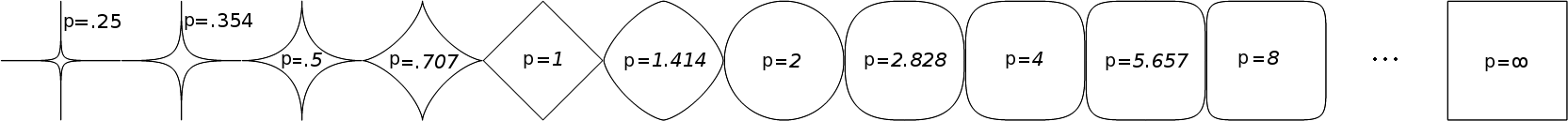# 正则化

## Lasso 回归

Lasso 算法（英语：least absolute shrinkage and selection operator，又译最小绝对值收敛和选择算子、套索算法）是一种同时进行特征选择和正则化（数学）的回归分析方法，旨在增强统计模型的预测准确性和可解释性，最初由斯坦福大学统计学教授 Robert Tibshirani 于 1996 年基于 Leo Breiman 的非负参数推断(Nonnegative Garrote, NNG)提出。

Lasso 回归：min $1/N\ast\sum_{i = 1}^{N}{(y_{i} -\omega^{T} x_{i})^{2} } + \lambda||\omega||_{1}$

## Ridge 回归

Ridge 回归：min $1/N\ast\sum_{i = 1}^{N}{(y_{i} -\omega^{T} x_{i})^{2} } + \lambda ||\omega||_{2}^{2}$

## 比较两者

Lasso 回归与 Ridge 回归有共同点，也有区别。

### 共同点

1. 线性回归出现的过拟合现象
2. 使用 Normal equation 求解时，解决 $(X^TX)$ 不可逆的问题。

### 区别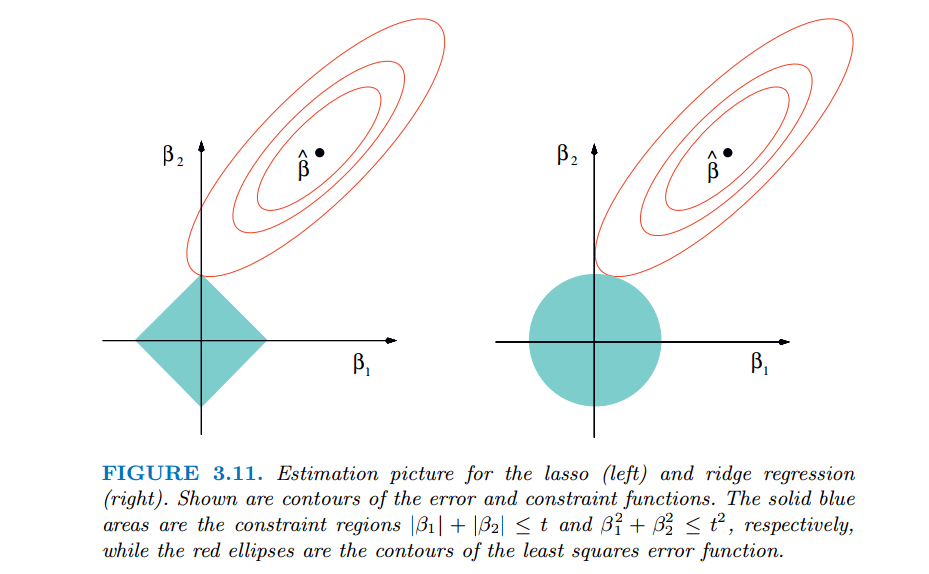# Reference

]]>
Stanford Machine Learning - 7 SVM http://luojinping.com/2018/04/14/Stanford-Machine-Learning-7-SVM/ 2018-04-14T10:33:50.000Z 2019-08-25T13:13:10.000Z SVM 定义

# 函数间隔和几何间隔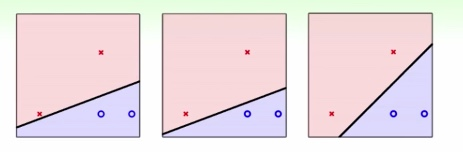## 符号定义

1. 在logstic回归中我们用0,1代表两个类, 现在我们改用-1,+1, 即 y∈{-1，-1}
2. 在logistic回归中, 我们的 g 是sigmoid函数, 现在改为:
g(z)=1, z>=0
g(z)=-1, z<0
3. 在logistic回归中, 我们的假设函数为 $h_θ(x)$, 现在改为, $h_\theta(x)$
$h_{x,b}(x)=g(w^{T}x+b)$, 其中w相当于$[θ_1,θ_2,θ_3,…θ_n]^T$, b 相当于 $θ_0$，即截距。

h_{x,b}

## 函数间隔(functional margin)

if $y^{(i)}=1$, want $(w^{T}x^{(i)}+b) \gg 0$ (>>代表远大于)
if $y^{(i)}=-1$, want $(w^{T}x^{(i)}+b) \ll 0$

## 几何间隔(geometric margin)

$w^{T}(x^{(i)} - \gamma^{(i)}\frac{w}{||w||})+b=0$

# 求解目标函数

## 使用对偶问题求解

SVM 中用到了高维映射，将线性不可分的问题映射为线性可分，且映射函数的具体形式无法提前确定，而往往使用核函数映射后，维度 w 会提升很多，甚至至无穷维。

### 优化公式

$\frac{∂L}{∂w}$ 和 $\frac{∂L}{∂b}$ 等于零，有：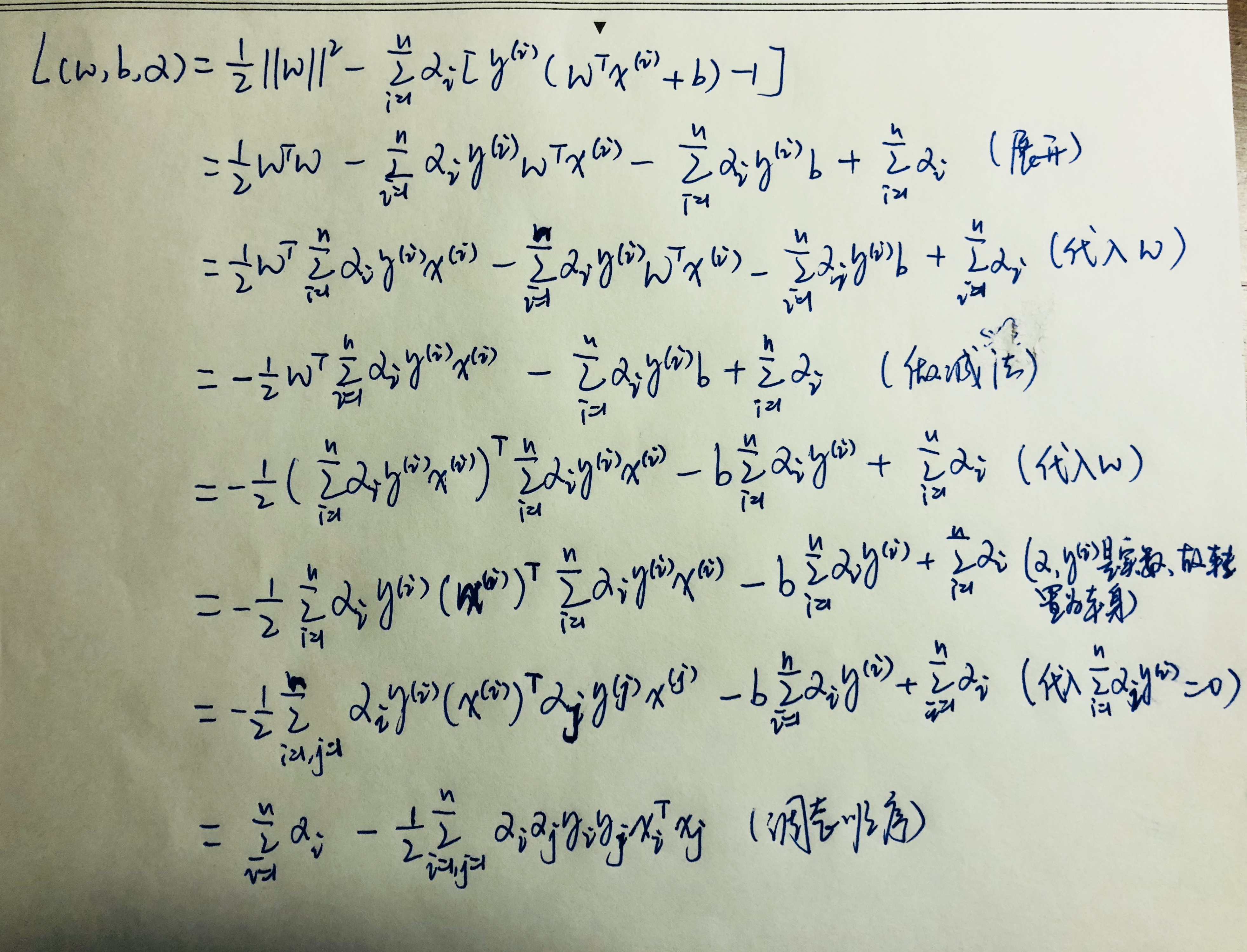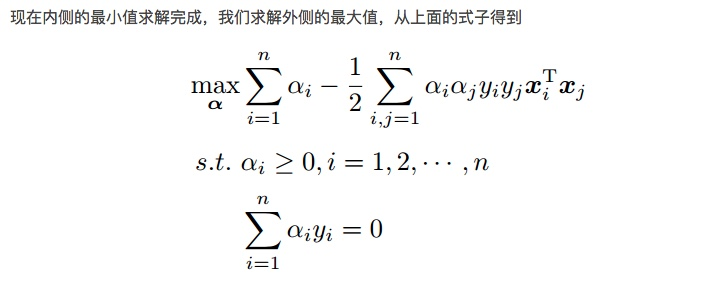## SMO 算法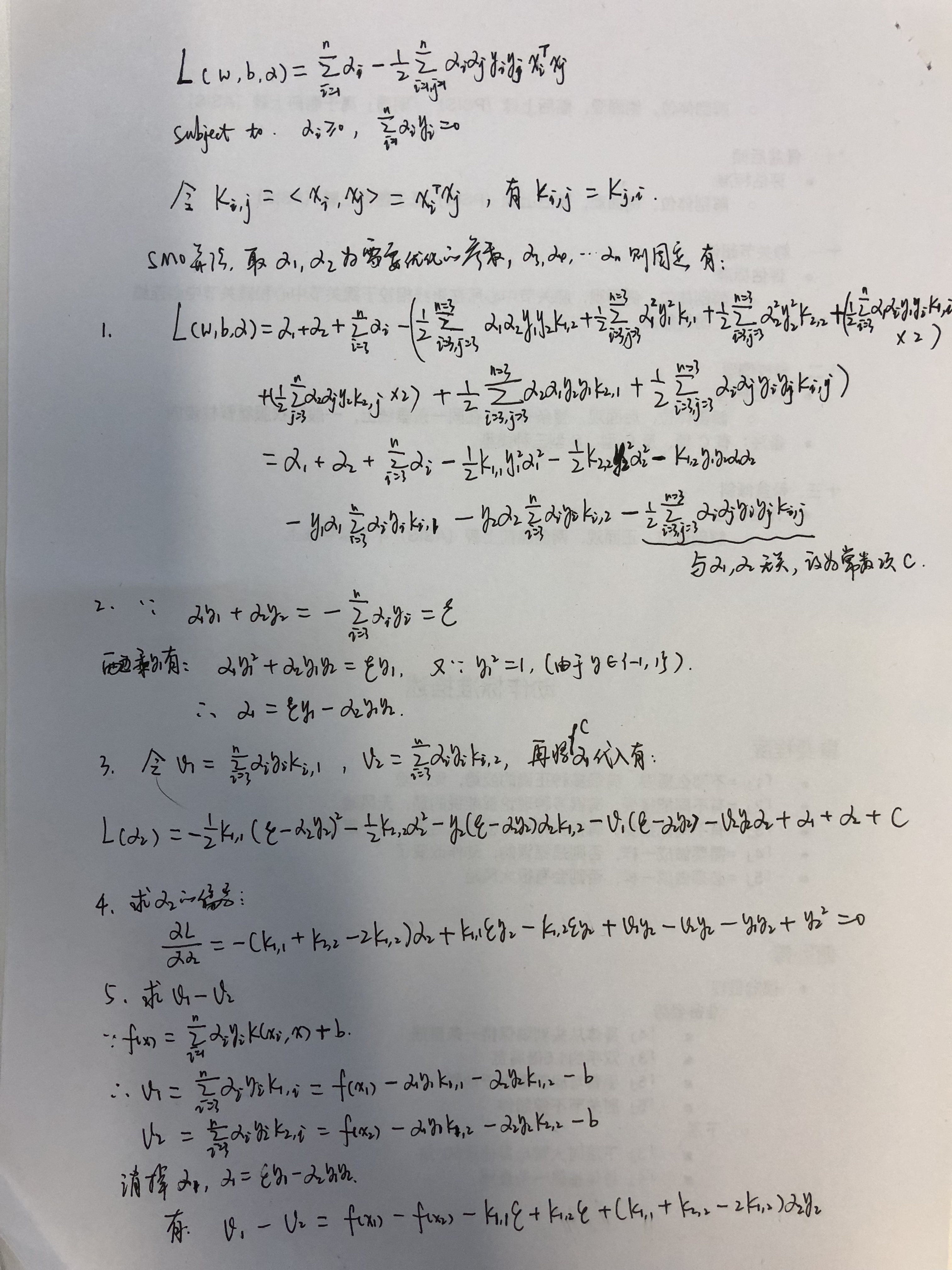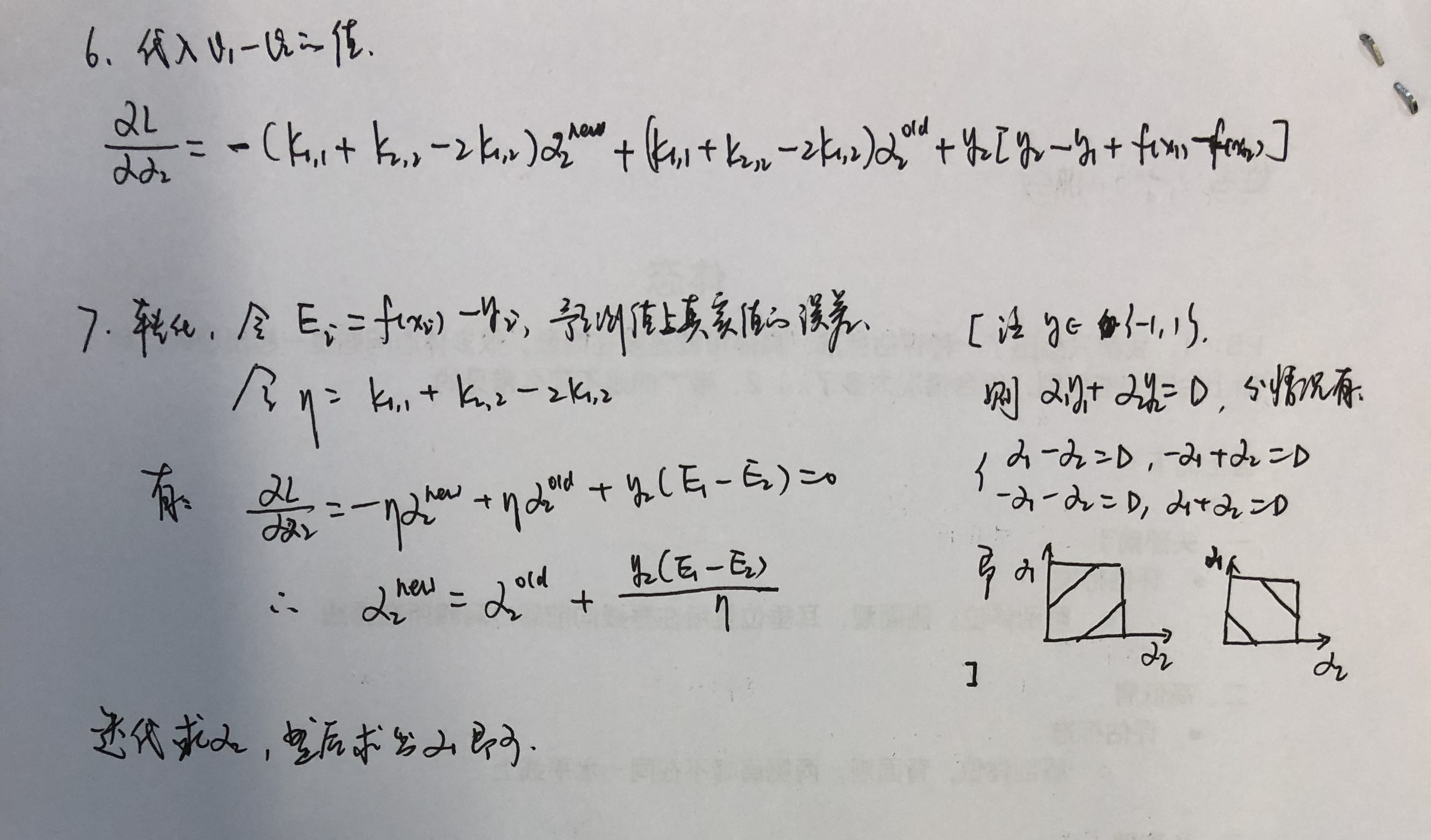# 核函数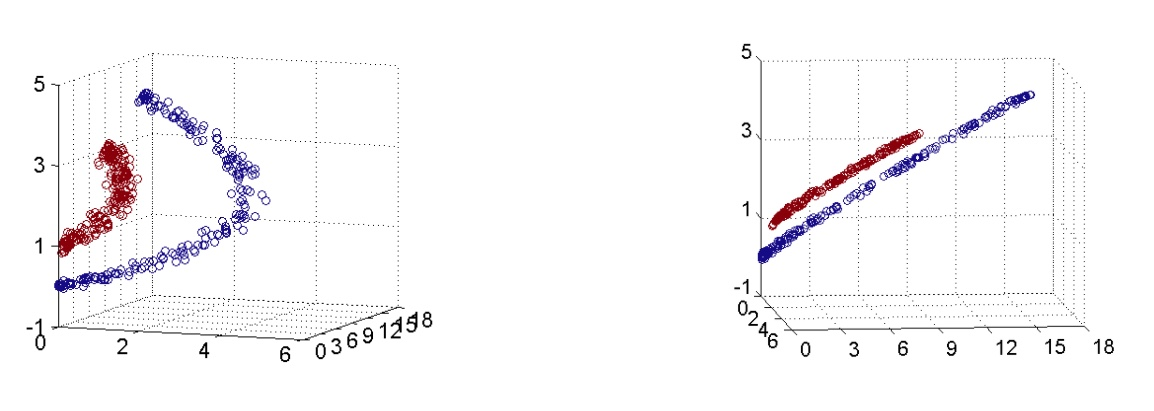# 总结 SVM

SVM 是神经网络出现之前最好的分类算法。求解 SVM 的过程也就是找到区分正负数据的最优超平面，所以引入了几何间隔的概念。而求解最大的几何间隔的问题即是在不等式约束条件下求解最优解的问题。这就引入了拉格朗日对偶问题，接着针对对偶问题求解，引入快速学习算法 SMO，最终找到超平面。

# SVM 优缺点

1. 可用于线性/非线性分类
2. 可以解决高维问题，即大型特征空间;
3. 泛化错误率低
4. 结果容易解释
5. 可以避免神经网络结构选择和局部极小点问题
6. SVM 尽量保持与样本间距离的性质导致它抗攻击的能力更强

1. 对参数调节和和函数的选择敏感，原始分类器不加修改仅适用于处理二分类问题
2. 在大规模训练样本下，效率不高
3. 对非线性问题有时很难找到一个合适的核函数
4. 解决多分类问题存在困难

# 附录

## 点到平面的距离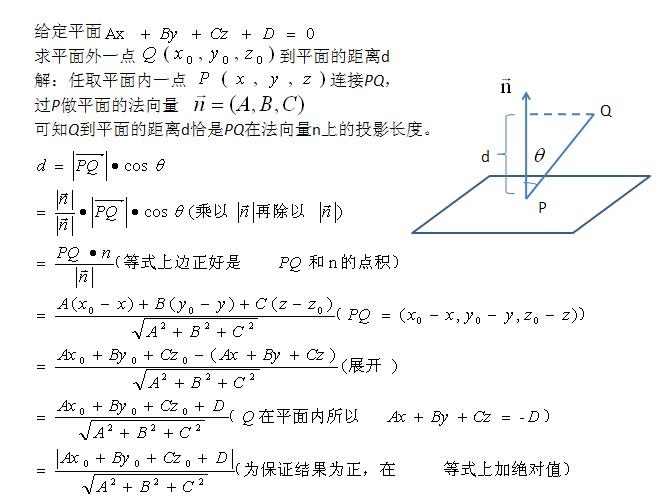$d$ 维空间中的超平面由下面的方程确定: $w^Tx+b=0$，其中，$w$ 与 $x$ 都是 $d$ 维列向量， $x=(x_1,x_2,…,x_d)^T$ 为平面上的点， $w=(w_1,w_2,…,w_d)^T$为平面的法向量。$b$ 是一个实数， 代表平面与原点之间的距离。

]]>

# 等式约束条件的优化问题

## 解法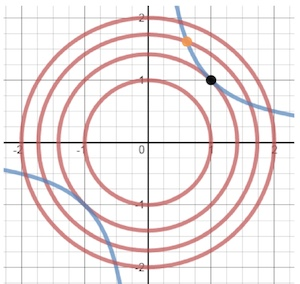$\frac{∂f}{∂x}=λ\frac{∂g}{∂x}$
$\frac{∂f}{∂y}=λ\frac{∂g}{∂y}$
$g(x,y)=0$

$\frac{∂L}{∂x}=\frac{∂f}{∂x}−λ\frac{∂g}{∂x}=0$
$\frac{∂L}{∂y}=\frac{∂f}{∂y}−λ\frac{∂g}{∂y}=0$
$\frac{∂L}{∂λ}=g(x,y)=0$

$L(x_1,…,x_n,λ_1,…,λ_k)=f(x1,…,xn)−\sum_{j=1}^{k}λ_jg_j(x_1,…,x_n)$

## 进一步理解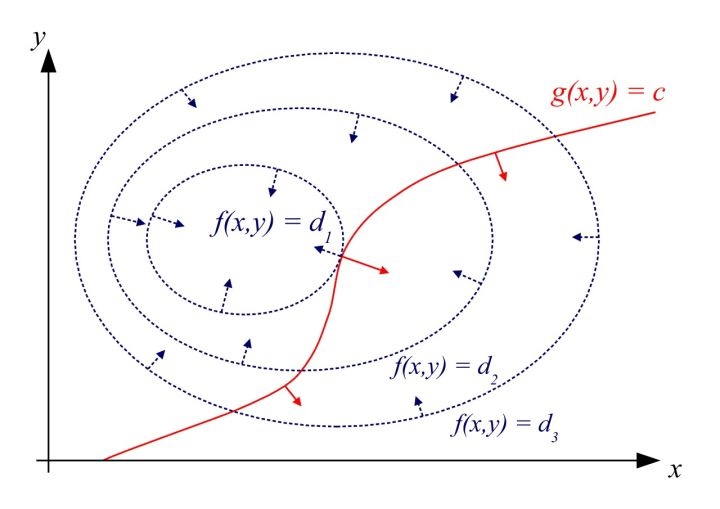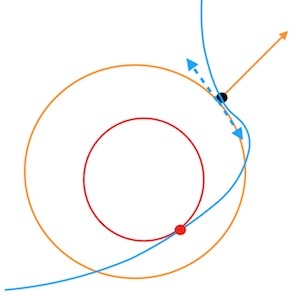# 凸优化

## 凸优化的意义

1. 其应用非常广泛，机器学习中很多优化问题都要通过凸优化来求解；
2. 在非凸优化中，凸优化同样起到重要的作用，很多非凸优化问题，可以转化为凸优化问题来解决；
3. 如上引用所述，凸优化问题可以看作是具有成熟求解方法的问题，而其他优化问题则未必。

## 对偶问题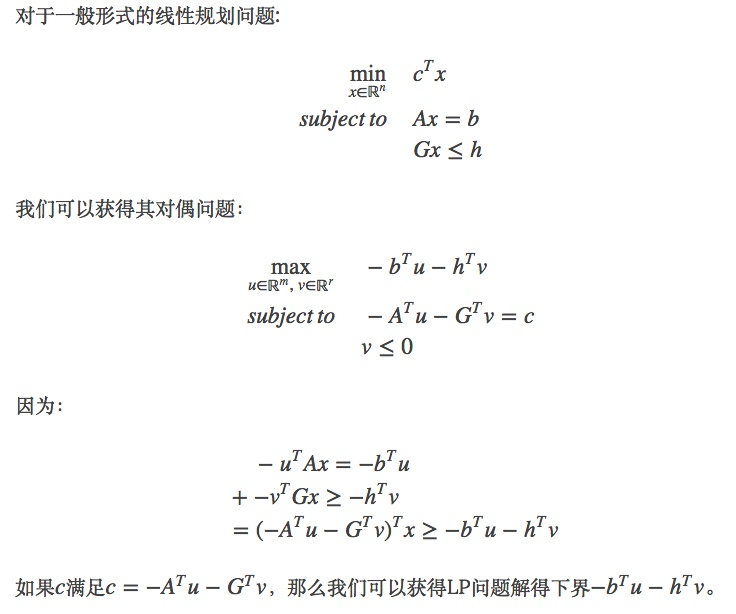# 不等式约束条件的优化问题

## 广义拉格朗日函数

$min.:f(x)$
$s.t.:$
$g_i(x) ≤ 0, i=1,2,…,p,$
$h_j(x) = 0, j=1,2,…,q,$
$x∈Ω⊂Rn$

## 定义原始问题最优解

1. 如果 $x$ 违反了约束条件，即 $g_i(x) > 0$ 或者 $h_j(x) \neq 0$，则容易存在 $μ_i -> +∞$，也容易存在 $λ_j$ 使得 $λ_jh_j(x) -> +∞$，这样显然 $\theta_p(x)$ 是没有最大值的。
2. 如果 $x$ 满足了约束条件，则 $\theta_p(x)={max}_{λ,μ:μ_i\ge0}L(x,λ,μ)={max}_{λ,μ:μ_i\ge0}f(x)=f(x)$，因为 ${max}_{λ,μ:μ_i\ge0}f(x)$ 与 $λ,μ$ 没有关系，所以其等于 $f(x)$。

## 对偶问题与原始问题的关系

1. 原始问题是凸优化问题的时候
2. 原始问题满足 Slater 条件（这一条还可以换成其他条件）
3. 最优解满足 KKT 条件

### Slater 条件

$p^∗=d^∗=L(x^∗,α^∗,β^∗)$

## KKT条件

KKT 条件(Karush-Kuhn-Tucker Conditions)，是指在满足一些有规则的条件下, 一个非线性规划(Nonlinear Programming)问题能有最优化解法的一个必要和充分条件，这是一个广义化拉格朗日乘数的成果。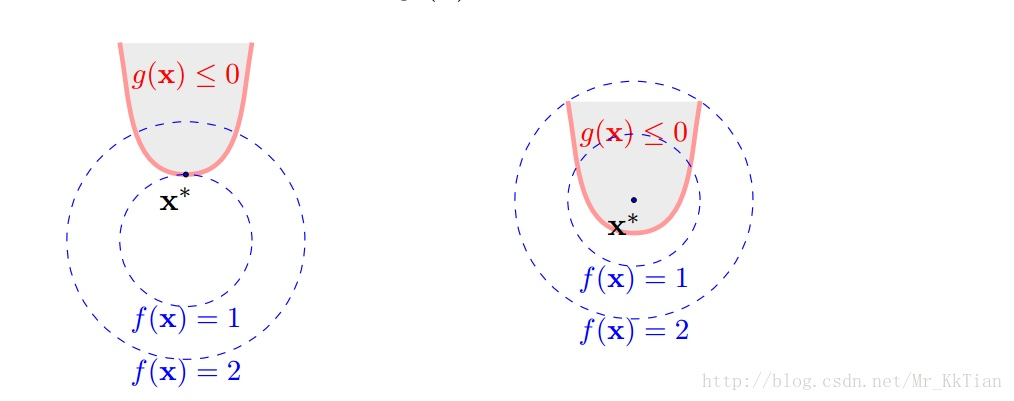1. 约束条件满足 $g_i(x^)≤0,i=1,2,…,p$, 以及 $h_j(x^)=0,j=1,2,…,q$
2. $∇f(x^)+\sum_{i=1}^{p}μ_i∇g_i(x^)+\sum_{j=1}^{q}λ_j∇h_j(x^*)=0$, 其中$∇$为梯度算子，也就是 L(x,u,λ) 对 x 求导为 0
3. $λ_j≠0$ 且不等式约束条件满足 $μ_i≥0$, $μ_ig_i(x^*)=0,i=1,2,…,p$，因为 $g(x)<=0$，如果要满足这个等式，必须 $μ_i=0$ 或者 $g(x)=0$

### KKT条件的推导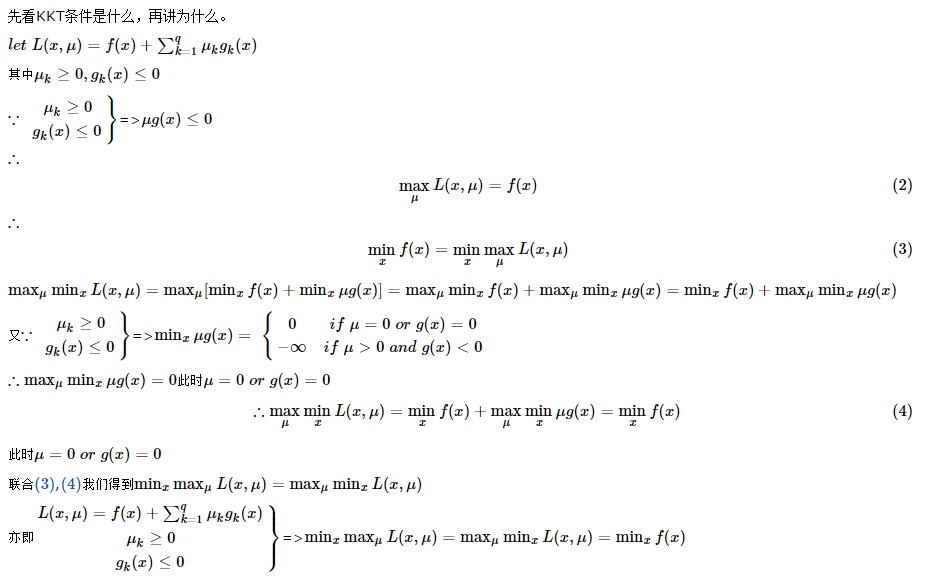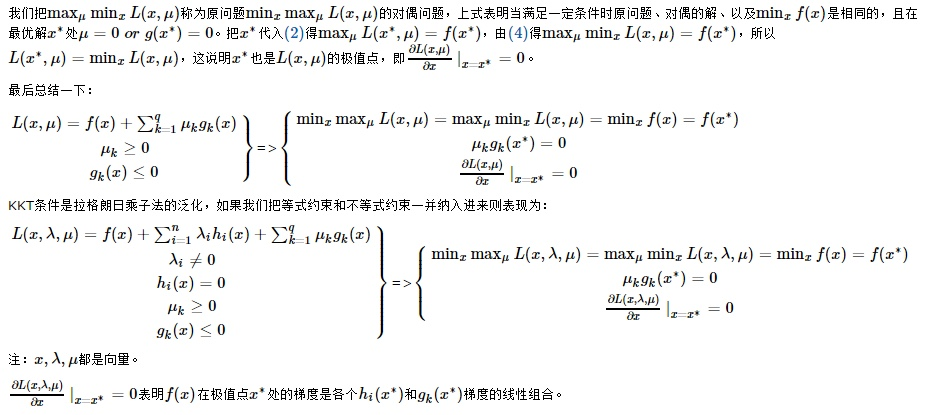## 总结

• 原问题是一个凸优化问题，则可以直接应用 KKT 条件来求解
• 原问题不是一个凸优化问题，则通过对偶问题转化为凸优化问题，在满足强对偶的条件下应用 KKT 条件求解

# Reference

]]>

$\displaystyle\lim_{x\to x_0}{f(x)}=f(x_0)$

# 导数

## 可导

（1）设 f(x)在 $x_0$ 及其附近有定义,则当 $\displaystyle\lim_{\Delta{x}\to0}{\frac{f(x_0+\Delta{x})-f(x_0)}{a}}$ 存在, 则称 f(x) 在 $x_0$ 处可导。
（2）若对于区间 (a,b) 上任意一点 (x，f(x)) 均可导，则称 f(x) 在 (a，b) 上可导。

# 微分

## 定义

$\Delta{y}=f(x+\Delta{x})-f(x)=f’(x)\Delta{x}+\alpha(\Delta{x})\Delta{x}$

## 可微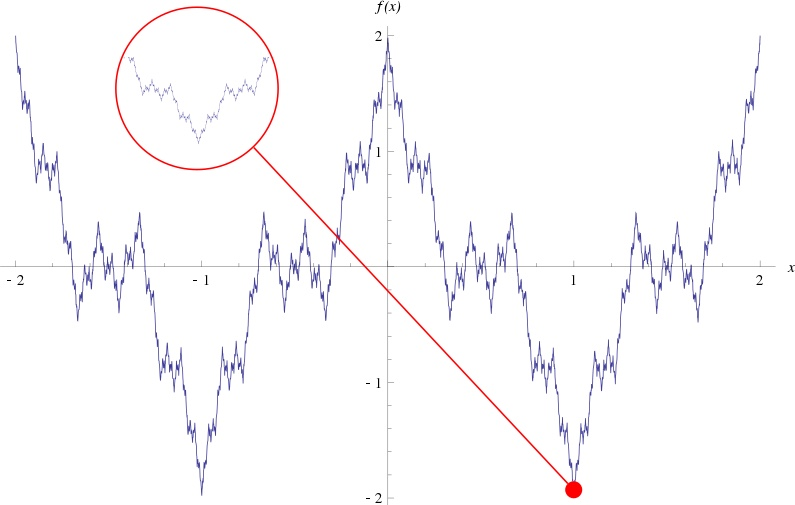# 连续，可导，可微的关系

• 一元函数：可导必然连续，连续推不出可导，可导与可微等价。
• 多元函数：可偏导与连续之间没有联系，也就是说可偏导推不出连续，连续推不出可偏导。
• 多元函数中可微必可偏导，可微必连续，可偏导推不出可微，但若一阶偏导具有连续性则可推出可微。

# 梯度

f(x+Δx,y+Δy)≈f(x,y)+∂f∂xΔx+∂f∂yΔy

# Reference

http://jermmy.xyz/2017/07/27/2017-7-27-understand-lagrange-multiplier/

]]>

# Reference

]]>
Jackson反序列化忽略为null的字段 http://luojinping.com/2017/08/19/Jackson反序列化忽略为null的字段/ 2017-08-19T15:02:30.000Z 2019-08-25T13:32:54.000Z 要解决的问题

json 反序列化 bean 时，当某个字段在 json 中为 null 时，使用 bean 中声明的默认值。

Person 类我们改造下：

# 解决方案

## JsonCreator 可行吗？

debug 过程：

com.fasterxml.jackson.databind.deser.SettableBeanProperty#deserialize，这个函数的实现是：

## 自定义 deserializer

Person 类改为：

]]>
<h1 id="要解决的问题"><a href="#要解决的问题" class="headerlink" title="要解决的问题"></a>要解决的问题</h1><p>json 反序列化 bean 时，当某个字段在 json 中为 null 时，使用 bean 中声明的默认值
lombok的AllArgsConstructor注解导致Jackson反序列化后丢失字段默认值 http://luojinping.com/2017/08/19/lombok的AllArgsConstructor注解导致Jackson反序列化后丢失字段默认值/ 2017-08-19T14:27:12.000Z 2019-08-25T13:25:50.000Z 要解决的问题

Person 类如下：

json:

# 解决过程

json 反序列化是从

## 根本原因

(补充自 liwei)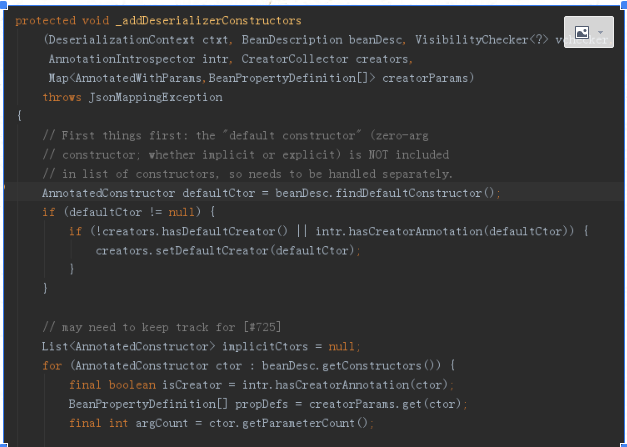# 结论

Lombok 的 @AllArgsConstructor 注解导致 Jackson 反序列化时调用了全参构造函数，将没有出现的字段都赋值为 null 了。

1. 不使用 @AllArgsConstructor
2. 使用 @AllArgsConstructor 但是不让其在全参构造函数上加入 ConstructorProperties 注解，声明方式改为 @AllArgsConstructor(suppressConstructorProperties = true)
]]>
<h1 id="要解决的问题"><a href="#要解决的问题" class="headerlink" title="要解决的问题"></a>要解决的问题</h1><p>希望在反序列化 json 到 bean 时，对于 json 中未出现的字段，在 bean 中赋上默认值。</